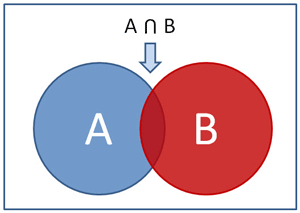# Learning Python with Advent of Code Walkthroughs

Dazbo's Advent of Code solutions, written in Python# Advent of Code 2022 - Day 4

## Problem Intro

We’re told the elves are cleaning space for their camp. Each elf has been assigned a range of camp areas to clean. The elves of sorted themselves into pairs and compared their cleanup assignments. Our input data contains one pair per line, like this:

``````2-4,6-8
2-3,4-5
5-7,7-9
2-8,3-7
6-6,4-6
2-6,4-8
``````

## Part 1

In how many assignment pairs does one range fully contain the other?

This is pretty trivial in Python. We just need to use set algebra.

First, we read each pair, and turn each x-y to a range. E.g. “2-4” becomes `[2,3,4]`. And then we store each range as a `set`, so that we can perform set algebra later.

``````def process_data(data: list[str]) -> list[set]:
""" Process data pairs.  Each line is a pair, with each item being an x-y range.
Convert each x-y range to a set, containing an expanded range of int values.
E.g. 2-4,6-8 -> [{2,3,4}{6,7,8}] """

pairs = [] # We want [{2,3,4}{6,7,8}]
for line in data:
this_pair = line.split(",") # E.g. ["2-4"]["6-8"]
assignments = []
for elf in this_pair:
start, end = list(map(int, elf.split("-")))  # E.g. 2, 4
assignments.append(set(range(start, end+1)))   # E.g. {2,3,4}

pairs.append(assignments)

return pairs
``````

A couple of notes on the code above:

• First we split the pairs at the comma.
• Then we split the “x-y” intervals at the “-“. This split returns `"x"` and `"y"`. But we want these as `int`, not as `str`, so we use the map function to perform the conversion.
• Then we convert our `x` and `y` boundaries to an actual `range`, convert the range to a `set` and store it.

Now we just need to compare the two ranges in each pair. We need to count where one range is equal to the other range, or completely includes the other range. This is so easy to do!

``````    includes_count = sum(1 for assn_1, assn_2 in pairs
if assn_1 == assn_2 or assn_1 < assn_2 or assn_2 < assn_1)
print(f"Part 1: Assigment inclusions = {includes_count}")
``````

Recall that when using sets, `<` means “is subset of”.

Note how I’m using list comprehension to count how many times our condition is satisfied. This pattern is described here.

## Part 2

Rather than counting complete inclusions, we now want to count all overlaps. I.e.

In how many assignment pairs do the ranges overlap?

We only need to add this…

``````    overlap_count = sum(1 for assn_1, assn_2 in pairs if assn_1 & assn_2)
print(f"Part 2: Assigment overlap = {overlap_count}")
``````

Recall that when using sets, `&` means “intersects with”. I.e. this gives us any overlap, which is exactly what we want!

## Results

The final code looks like this:

``````from pathlib import Path
import time

SCRIPT_DIR = Path(__file__).parent
# INPUT_FILE = Path(SCRIPT_DIR, "input/sample_input.txt")
INPUT_FILE = Path(SCRIPT_DIR, "input/input.txt")

def main():
with open(INPUT_FILE, mode="rt") as f:

pairs = process_data(data)

includes_count = sum(1 for assn_1, assn_2 in pairs
if assn_1 == assn_2 or assn_1 < assn_2 or assn_2 < assn_1)
print(f"Part 1: Assigment inclusions = {includes_count}")

overlap_count = sum(1 for assn_1, assn_2 in pairs if assn_1 & assn_2)
print(f"Part 2: Assigment overlap = {overlap_count}")

def process_data(data: list[str]) -> list[set]:
""" Process data pairs.  Each line is a pair, with each item being an x-y range.
Convert each x-y range to a set, containing an expanded range of int values.
E.g. 2-4,6-8 -> [{2,3,4}{6,7,8}] """

pairs = [] # We want [[2,3,4][6,7,8]]
for line in data:
this_pair = line.split(",") # E.g. ["2-4"]["6-8"]
assignments = []
for elf in this_pair:
start, end = list(map(int, elf.split("-")))  # E.g. 2, 4
assignments.append(set(range(start, end+1)))   # E.g. {2,3,4}

pairs.append(assignments)

return pairs

if __name__ == "__main__":
t1 = time.perf_counter()
main()
t2 = time.perf_counter()
print(f"Execution time: {t2 - t1:0.4f} seconds")
``````

And the output looks like this:

``````Part 1: Assigment inclusions = 500
Part 2: Assigment overlap = 815
Execution time: 0.0066 seconds
``````